Lecture 12: Vibrational Spectroscopy of Diatomic Molecules

•• Contributed by Delmar Larsen
• Founder and Director at Libretexts

Recap of Lecture 11

Last lecture continued the discussion of vibrations into the realm of quantum mechanics. We reviewed the classical picture of vibrations including the classical potential, bond length, and bond energy. We then introduced the quantum version using the harmonic oscillator as an approximation of the true potential. This involves constructing a Hamilonian with a parabolic potential. Solving the resulting (time-independent) Schrödinger equation to obtain the eigeinstates, energies, and quantum numbers (v) results is beyond this course, so they are given. Key aspect of these solution are the fundamental frequency and zero-point energy.

Vibrations

The motion of two particles in space can be separated into translational, vibrational, and rotational motions.Different ways of visualizing the 6 degrees of freedom of a diatomic molecule. (CC BY-NC-SA; anonymous by request)

IR spectroscopy which has become so useful in identification, estimation, and structure determination of compounds draws its strength from being able to identify the various vibrational modes of a molecule. A complete description of these vibrational normal modes, their properties and their relationship with the molecular structure is the subject of this article.

Degree of freedom is the number of variables required to describe the motion of a particle completely. For an atom moving in 3-dimensional space, three coordinates are adequate so its degree of freedom is three. Its motion is purely translational. If we have a molecule made of N atoms (or ions), the degree of freedom becomes 3N, because each atom has 3 degrees of freedom. Furthermore, since these atoms are bonded together, all motions are not translational; some become rotational, some others vibration.

The degrees of vibrational modes for linear molecules can be calculated using the formula:

$3N-5 \label{1}$

The degrees of freedom for nonlinear molecules can be calculated using the formula:

$3N-6 \label{2}$

$$n$$ is equal to the number of atoms within the molecule of interest. The following procedure should be followed when trying to calculate the number of vibrational modes:

1. Determine if the molecule is linear or nonlinear (i.e. Draw out molecule using VSEPR). If linear, use Equation \ref{1}. If nonlinear, use Equation \ref{2}
2. Calculate how many atoms are in your molecule. This is your $$N$$ value.
3. Plug in your $$N$$ value and solve.
Atoms (very symmetric) Linear molecules (less symmetric) Non-linear molecules (most unsymmetric)
Translation (x, y, and z) 3 3 3
Rotation (x, y, and z) 0 2 3
Vibrations 0 3N − 5 3N − 6
Total (including Vibration) 3 3N 3N

Example 1: Water• The Symmetric Stretch (Example shown is an H2O molecule at 3685 cm-1)
• The Asymmetric Stretch (Example shown is an H2O molecule at 3506 cm-1)
• Bend (Example shown is an H2O molecule at 1885 cm-1)

A linear molecule will have another bend in a different plane that is degenerate or has the same energy. This accounts for the extra vibrational mode.

Example 2: Carbon DioxideExample 3: The Methylene Group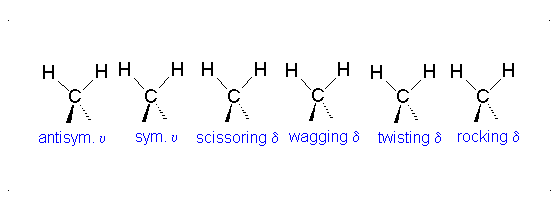It is important to note that there are many different kinds of bends, but due to the limits of a 2-dimensional surface it is not possible to show the other ones.

The frequency of these vibrations depend on the inter atomic binding energy which determines the force needed to stretch or compress a bond.

Properties of a Molecular Bond

What do we know about bonds from general chemistry?

1. Breaking a bond always requires energy and hence making bonds always release energy.
2. Bond length
3. Bond energy (or enthalpy or strength)

The potential energy of a system of two atoms depends on the distance between them. At large distances the energy is zero, meaning “no interaction”. At distances of several atomic diameters attractive forces dominate, whereas at very close approaches the force is repulsive, causing the energy to rise. The attractive and repulsive effects are balanced at the minimum point in the curve.The internuclear distance at which the potential energy minimum occurs defines the bond length. This is more correctly known as the equilibrium bond length, because the two atoms will always vibrate about this distance.

Bond lengths depend mainly on the sizes of the atoms, and secondarily on the bond strengths, the stronger bonds tending to be shorter. Bonds involving hydrogen can be quite short; The shortest bond of all, H–H, is only 74 pm. Multiply-bonded atoms are closer together than singly-bonded ones; this is a major criterion for experimentally determining the multiplicity of a bond. This trend is clearly evident in the above plot which depicts the sequence of carbon-carbon single, double, and triple bonds.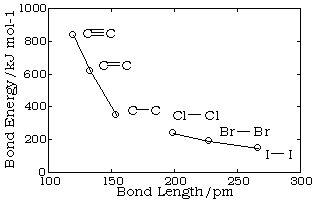In general, the stronger the bond, the smaller will be the bond length.

Reduced mass (Converting two atoms moving into one)

The internal motions of vibration and rotation for a two-particle system can be described by a single reduced particle with a reduced mass $$μ$$ located at $$r$$. For a diatomic molecule, In the below figure, the vector $$\vec{r}$$ corresponds to the internuclear axis. The magnitude or length of $$r$$ is the bond length, and the orientation of $$r$$ in space gives the orientation of the internuclear axis in space. Changes in the orientation correspond to rotation of the molecule, and changes in the length correspond to vibration. The change in the bond length from the equilibrium bond length is the vibrational coordinate for a diatomic molecule.The diagram shows the coordinate system for a reduced particle. $$R_1$$ and $$R_2$$ are vectors to $$m_1$$ and $$m_2$$. $$R$$ is the resultant and points to the center of mass. (b) Shows the center of mass as the origin of the coordinate system, and (c) expressed as a reduced particle.

The Classical Harmonic Oscillator

Simple harmonic oscillators about a potential energy minimum can be thought of as a ball rolling frictionlessly in a dish (left) or a pendulum swinging frictionlessly back and forth. The restoring forces are precisely the same in either horizontal direction.Simple image of a ball oscillating in a potential. Image used with permission from Wikipedia.

Recall that the Hamiltonian operator $$\hat{H}$$ is the summation of the kinetic and potential energy in a system. There are several ways to approximate the potential function $$V$$, but the two main means of approximation are done by using a Taylor series expansion, and the Morse Potential. The vibration of a diatomic is akin to an oscillating mass on a spring.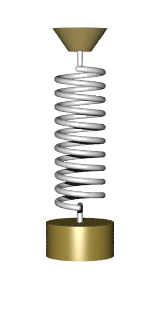An undamped spring–mass system undergoes simple harmonic motion. Image used with permission from Wikipedia.

The classical forces in chemical bonds can be described to a good approximation as spring-like or Hooke's law type forces. This is true provided the energy is not too high. Of course, at very high energy, the bond reaches its dissociation limit, and the forces deviate considerably from Hooke's law. It is for this reason that it is useful to consider the quantum mechanics of a harmonic oscillator.

We will start in one dimension. Note that this is a gross simplification of a real chemical bond, which exists in three dimensions, but some important insights can be gained from the one-dimensional case. The Hooke's law force is

$F(x)=-k(x-x_0)$

where $$k$$ is the spring constant. This force is derived from a potential energy

$V(x)=\dfrac{1}{2}k(x-x_0)^2$

Let us define the origin of coordinates such that $$x_0 =0$$. Then the potential energy

$\color{red} V(x)=\dfrac{1}{2}kx^2$

If a particle of mass $$m$$ is subject to the Hooke's law force, then its classical energy is

$\dfrac{p^2}{2m}+\dfrac{1}{2}kx^2 =E$

Thus, we can set up the Schrödinger equation:

$\left [ -\dfrac{(\hbar)^2}{2m}\dfrac{d^2}{dx^2}+\dfrac{1}{2}kx^2 \right ]\psi (x)=E\psi (x)$

In this case, the Hamiltonian is

$\hat{H}=-\dfrac{(\hbar)^2}{2m}\dfrac{d}{dx^2}+\dfrac{1}{2}kx^2$

Since $$x$$ now ranges over the entire real line $$x\epsilon (-\infty ,\infty)$$, the boundary conditions on $$\psi (x)$$ are conditions at $$x=\pm \infty$$. At $$x= \pm \infty$$, the potential energy becomes infinite. Therefore, it must follow that as $$x \rightarrow \pm \infty$$, $$\psi (x)\rightarrow 0$$. Hence, we can state the boundary conditions as $$\psi (\pm \infty)=0$$.

Solving this differential equation is not an easy task, so we will not attempt to do it. Here, we simply quote the allowed energies and some of the wave functions. The allowed energies are characterized by a single integer $$v$$, which can be $$0,1,2,...$$ and take the form

$\color{red} E_v =\left ( v+\dfrac{1}{2} \right )h\nu_1 \label{BigEq}$

where $$\nu$$ is the frequency of the oscillation (of a single mass on a spring):

$\nu_{1} =\dfrac{1}{2\pi}\sqrt{\dfrac{k}{m}}$

$$\nu_1$$ is the fundamental frequency of the mechanical oscillator which depends on the force constant of the spring and a single mass of the attached (single) body and independent of energy imparted on the system. when there are two masses involved in the system (e.g., a vibrating diatomic), then the mass used in Equation $$\ref{BigEq}$$ becomes is a reduced mass:

$\color{red} \mu = \dfrac{m_1 m_2}{m_1+m_2} \label{14}$

The fundamental vibrational frequency is then rewritten as

$\nu = \dfrac{1}{2\pi} \sqrt{\dfrac{k}{\mu}} \label{15}$

Do not confuse $$v$$ the quantum number for harmonic oscillator with $$\nu$$ the fundamental frequency of the vibration

The natural frequency $$\nu$$ can be converted to angular frequency $$\omega$$ via

$\omega = 2\pi \nu$

Then the energies in Equation $$\ref{BigEq}$$ can be rewritten in terms of the fundamental angular frequency as

$\color{red} E_v =\left ( v+\dfrac{1}{2} \right )\hbar \omega \label{BigEq2}$

Now the eigenstates

Now we can define the parameter (for convenience)

$\alpha =\dfrac{\sqrt{km}}{\hbar}=\dfrac{m\omega}{\hbar}=\dfrac{4\pi ^2m\nu}{h}$

the first few wave functions are

\begin{align*}\psi_0 (x) &= \left ( \dfrac{\alpha}{\pi} \right )^{1/4}e^{-\alpha x^2 /2}\\ \psi_1(x) &= \left ( \dfrac{4\alpha ^3}{\pi} \right )^{1/4}xe^{-\alpha x^2 /2}\\ \psi_2 (x) &= \left ( \dfrac{\alpha}{4\pi} \right )^{1/4}(2\alpha x^2 -1)e^{-\alpha x^2/2}\\ \psi_3 (x) &= \left ( \dfrac{\alpha ^3}{9\pi} \right )^{1/4}(2\alpha x^3 -3x)e^{- \alpha x^2 /2}\end{align*}

You should verify that these are in fact solutions of the Schrödinger equation by substituting them back into the equation with their corresponding energies. The figure below shows these wave functionsThe harmonic oscillator wavefunctions describing the four lowest energy states.

The figure below shows these wave functions and the corresponding probability densities: $$p_n (x)=\psi_{n}^{2}(x)$$: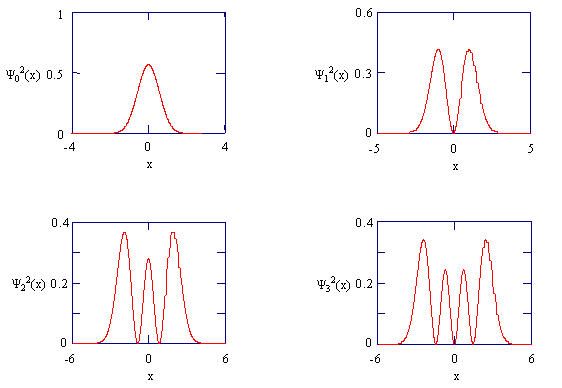The probability densities for the four lowest energy states of the harmonic oscillator.

Note that in contrast to a particle in an infinite high box, $$x\epsilon (-\infty ,\infty)$$, so the normalization conditionfor each eignestate is

$\int_{-\infty}^{\infty}\psi_{n}^{2}(x)dx=1$

Despite this, because the potential energy rises very steeply, the wave functions decay very rapidly as $$|x|$$ increases from 0 unless $$n$$ is very large. This is discussed as tunneling elsewhere.

Another way to show solutions to harmonic oscillators. (left) Wavefunction representations for the first eight bound eigenstates, n = 0 to 7. The horizontal axis shows the position x. Note: The graphs are not normalized, and the signs of some of the functions differ from those given in the text. (right) Corresponding probability densities.Images used with permission (CC BY-SA 3.0; AllenMcC.)

Recap of Lecture 10

Last lecture addressed three aspects. The first is the introduction of the commutator which is meant to evaluate is two operators commute (a property used extensively in basic algebra courses). Not every pair of operators will commute meaning the order of operations matter. The second aspect is to redefine the Heisenberg Uncertainty Principle now within the context of commutators. Now, we can identify if any two quantum measurements (i.e., eigenvalues of specific operators) will require the Heisenberg Uncertainty Principle to be addressed when simultaneously evaluating them. The third aspect of the lecture was the introduction of vibrations, including how many vibrations a molecule can he (depending on linearity) and the origin of this. The solutions to the harmonic oscillator potential were qualitatively shown (via Java application) with an emphasis of the differences between this model system and the particle in the box (important).

Atoms (very symmetric) Linear molecules (less symmetric) Non-linear molecules (most unsymmetric)
Translation (x, y, and z) 3 3 3
Rotation (x, y, and z) 0 2 3
Vibrations 0 3N − 5 3N − 6
Total (including Vibration) 3 3N 3N

Quantum Mechanical Vibrations

The simplified potential discussed in general chemistry is the potential energy function used in constructing the Hamiltonian. From solving the Schrodinger equations, we get eigenfunctions, eigenvalues (energies) and quantum numbers. Combining these on the potential like we did for the particle in a box give a more detailed (quantum) pictureGeneral Potential (not am approximation) of a vibration with associated eigenenergies.

Zero-point energy

Zero-point energy is the lowest possible energy that a quantum mechanical system may have,i.e. it is the energy of the system's ground state. The uncertainty principle states that no object can ever have precise values of position and velocity simultaneously.

For the particle in the 1D box of length $$L$$, with energies

$E_n = \dfrac{h^2 n^2 }{8m L^2}$

the zero-point energy ($$n=1$$) is

$E_{ZPE} = \dfrac{h^2}{8m L^2}$

For the Harmonic Oscillator with energies

$E_v =\left ( v+\dfrac{1}{2} \right )h\nu$

the zero-point energy ($$v=0$$) is

$E_v = \dfrac{h\nu}{2} = \dfrac{\hbar\omega }{2}$

Many people have make cookie ideas of tapping into the zero-point energy to drive our economy. This is a silly idea and impossible, since this energy cannot never be tapped (see https://van.physics.illinois.edu/qa/...ng.php?id=1256 for more discussion

Infrared Spectroscopy

Infrared (IR) spectroscopy is one of the most common and widely used spectroscopic techniques employed mainly by inorganic and organic chemists due to its usefulness in determining structures of compounds and identifying them. Chemical compounds have different chemical properties due to the presence of different functional groups. A molecule composed of n-atoms has 3n degrees of freedom, six of which are translations and rotations of the molecule itself. This leaves 3N-6 degrees of vibrational freedom (3N-5 if the molecule is linear). Vibrational modes are often given descriptive names, such as stretching, bending, scissoring, rocking and twisting. The four-atom molecule of formaldehyde, the gas phase spectrum of which is shown below, provides an example of these terms.

The spectrum of gas phase formaldehyde, is shown below.Gas Phase Infrared Spectrum of Formaldehyde, $$H_2C=O$$

Characteristic normal modes (vibrations) in formaldehyde

• $$CH_2$$ Asymmetric Stretch
• $$CH_2$$ Symmetric Stretch
• $$C=O$$ Stretch
• $$CH_2$$ Scissoring
• $$CH_2$$ Rocking
• $$CH_2$$ Wagging

The exact frequency at which a given vibration occurs is determined by the strengths of the bonds involved and the mass of the component atoms.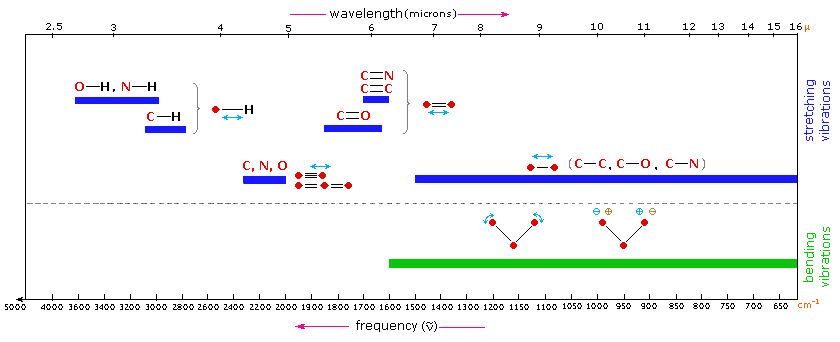Transition Moment Integrals gives Selection rules

Spectroscopy is a matter-light interaction. You first need to know the results of the Schrödinger equation of a specific system. This include both eigenstates (wavefuctions), eigenvalues (energies), and quantum numbers and You need to understand how to couple the eigenstates with electromagnetic radiation. This is done via the transition moment integral

$\langle \psi_i | \hat{M}| \psi_f \rangle \label{26}$

The transition moment integral gives information about the probability of a transition occurring. For IR of a single harmonic oscillator, $$\hat{M}$$ can be set to $$x$$. A more detailed discussion will be presented later. So the probability for a transiton in HO is $P_{i \rightarrow f} = \langle \psi_i | x | \psi_f \rangle$

• From Equation $$\ref{26}$$ comes general rules for absorption. For IR, the transition is allowed only if the molecule has a changing dipole moment.
• From Equation $$\ref{26}$$ comes selection rules (what possible transitions are allowed). For IR this results in $$\Delta v = \pm 1$$.

The vibration must change the molecular dipole moment to have a non-zero (electric) transition dipole moment. Molecules CAN have a zero net dipole moment, yet STILL UNDERGO transitions when stimulated by infrared light.

Dipole Moments (rehash from gen chem)

When two electrical charges, of opposite sign and equal magnitude, are separated by a distance, a dipole is established. The size of a dipole is measured by its dipole moment ($$\mu$$). Dipole moment is measured in Debye units, which is equal to the distance between the charges multiplied by the charge (1 Debye equals $$3.34 \times 10^{-30}\; C\, m$$). The dipole moment of a molecule can be calculated by Equation $$\ref{d1}$$:

$\vec{\mu} = \sum_i q_i \, \vec{r}_i \label{d1}$

where

• $$\vec{\mu}$$ is the dipole moment vector
• $$q_i$$ is the magnitude of the $$i^{th}$$ charge, and
• $$\vec{r}_i$$ is the vector representing the position of $$i^{th}$$ charge.

The dipole moment acts in the direction of the vector quantity. An example of a polar molecule is $$H_2O$$. Because of the lone pair on oxygen, the structure of H2O is bent (via VEPSR theory), which that the vectors representing the dipole moment of each bond do not cancel each other out. Hence, water is polar.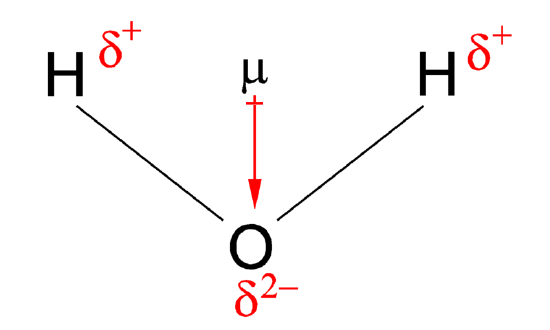Dipole moment of water. The convention in chemistry is that the arrow representing the dipole moment goes from positive to negative. In physics, the opposite is used.

Example $$\PageIndex{1}$$:

Which molecules absorb IR radiation?

$$\ce{O2}$$

No: Vibration does not change the dipole moment of the molecule due to symmetry.

$$\ce{HCl}$$

Yes: Vibration does change the dipole moment of the molecule since there is a different in electronegativity so the distance between the two atoms affects the dipole moment (Equation \ref{d1}).

$$\ce{H2}$$

No: Vibration does not change the dipole moment of the molecule due to symmetry.

$$\ce{Cl2}$$

No: Vibration does not change the dipole moment of the molecule due to symmetry.

$$\ce{CO2}$$

Yes.: A vibration does change the dipole moment of the molecule since there is a different in electronegativity so the distance between the two atoms affects the dipole moment. This is not the symetric stretch, but the other modes.Energies of Harmonic Oscillators and IR Transitions

Using the harmonic oscillator and wave equations of quantum mechanics, the energy can be written in terms of the spring constant and reduced mass as

$E = \left(v+\dfrac{1}{2}\right) \dfrac{h}{2\pi} \sqrt{\dfrac{k}{\mu}} \label{16}$

where h is Planck's constant and v is the vibrational quantum number and ranges from 0,1,2,3.... infinity.

$E = \left(v+\dfrac{1}{2}\right)h \nu \label{17}$

where $$\nu$$ is the vibrational frequency. Transitions in vibrational energy levels can be brought about by absorption of radiation, provided the energy of the radiation exactly matches the difference in energy levels between the vibrational quantum states and provided the vibration causes a change in dipole moment. This can be expressed as

${\triangle E} = h\nu = \dfrac{h}{2\pi} \sqrt{\dfrac{k}{\mu}} \label{18}$

At room temperature, the majority of molecules are in the ground state $$v = 0$$, from the equation above

$E_o = \dfrac{1}{2}hv \label{19}$

when a molecule absorbs energy, there is a promotion to the first excited state

$E_1 = \dfrac{3}{2} hv \label{20}$

$\left(\dfrac{3}{2} h\nu_m - \dfrac{1}{2} hv_m \right) = hv \label{21}$

The frequency of radiation $$\nu$$ that will bring about this change is identical to the classical vibrational frequency of the bond and it can be expressed as

$\color{ref} E_{radiation} = hv = {\triangle E} = hv_m = \dfrac{h}{2\pi} \sqrt{\dfrac{k}{\mu}} \label{22}$

The above equation can be modified so that the radiation can be expressed in wavenumbers

$\color{red} \widetilde{\nu} = \dfrac{1}{2\pi c} \sqrt{\dfrac{k}{\mu}} \label{23}$

where

• $$c$$ is the velocity of light (cm s-1) and
• $$\widetilde{\nu}$$ is the wave number of an absorption maximum (cm-1)Corresponding probability densities. Image used with permission (Public domain; Allen McC.)

Java simulation of particles in boxes :https://phet.colorado.edu/en/simulation/bound-states

Selection Rules

Photons can be absorbed or emitted, and the harmonic oscillator can go from one vibrational energy state to another. Which transitions between vibrational states are allowed? If we take an infrared spectrum of a molecule, we see numerous absorption bands, and we need to relate these bands to the allowed transitions involving different normal modes of vibration.

The selection rules are determined by the transition moment integral.

$\mu_T = \int \limits _{-\infty}^{\infty} \Psi_{v'}^* (Q) \hat {\mu} (Q) \Psi _v (Q) dQ = \langle \Psi_{v'} |\hat {\mu} |\Psi _v \rangle \label {6.6.1}$

To evaluate this integral we need to express the dipole moment operator, $$\hat {\mu}$$, in terms of the magnitude of the normal coordinate $$Q$$. Evaluating the integral in Equation $$\ref{6.6.1}$$ can be difficult depending on the complexity of the wavefunctions used. We can often (although not always) take advantage of the symmetries of the waveunfction (and $$\hat {\mu}$$ too) to make things easlier.Figure: (left) $$f(x) = x^2$$ is an example of an even function. (right) $$f(x) = x^3$$ is an example of an odd function. Images used with permission from Wikipedia.

While functions exhibit this symmetry, the product of functions inherent the symmetries of the constituent components via a "product table." The one below is in term of odd/even symmetry, but as you will learn in other classes (especially group theories), there are several symmetries that 3D objects have. The product tables constructed that take into account all such symmetries are more complicated.

Product table Odd Function (anti-symmetric) Even Function (symmetric) No symmetry (neither odd nor even)
Odd Function (anti-symmetric) Even Function (symmetric) Odd Function (anti-symmetric) who knows
Even Function (symmetric) Odd Function (anti-symmetric) Even Function (symmetric) who knows
No symmetry (neither odd nor even) who knows who knows who knows

These symmetries are important since the integrand of an integral (over all space) of an odd function is ALWAYS zero. So you do no need to solve it.

And now the Harmonic Oscillator Wavefunctions

Because of the association of the wavefunction with a probability density, it is necessary for the wavefunction to include a normalization constant, $$N_v$$.

$N_v = \dfrac {1}{(2^v v! \sqrt {\pi} )^{1/2}} \label {5.6.15}$

The final form of the harmonic oscillator wavefunctions is the product of thee terms:

$\color{red} | \Psi _v (x) \rangle = N_v H_v (x) e^{-x^2/2} \label {5.6.16}$

The first few physicists' Hermite polynomials are:

$$H_0(x)=1\,$$

$$H_1(x)=2x\,$$

$$H_2(x)=4x^2-2\,$$

$$H_3(x)=8x^3-12x\,$$

$$H_4(x)=16x^4-48x^2+12\,$$

$$H_5(x)=32x^5-160x^3+120x\,$$

The symmetry of the Harmonic Oscillator wavefunctions is dictated by the Hermite Polynomials• Which levels are even functions and which are odd functions?
• Without calculating an integral, what is $$\langle x\rangle$$?